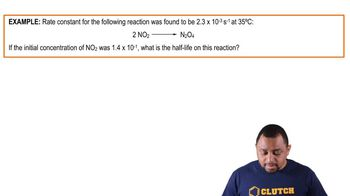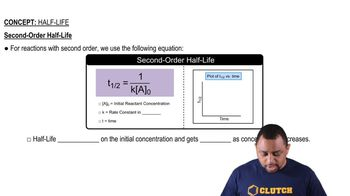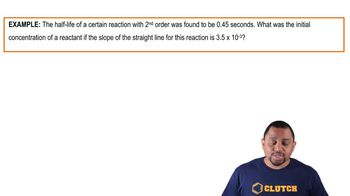Start typing, then use the up and down arrows to select an option from the list.# Half-Life Concept 1

Jules Bruno
173views
now half life is just the time it takes to lose half of a reacting to decay or decomposed in a certain time period. Now here the way half by function really depends on the order. Remember we have zeroth order, first order and second order. Now if we're talking about zero order, we're gonna say for zero order reactions, we use the following equation for half life here half life equals the initial concentration of our reactant divided by two times K. Here a sub zero is again our initial reacting concentration K equals our rate constant. Remember for for um for zero order processes we'd say that its units of polarity times time, inverse here, T in this case is time. But when we have t half that really stands for half life. Now if we take a look at this we'd say what happens to half life? If our initial concentration gets blank as concentration decreases. Well here we're going to say that since half life is part of the equation, we're going to say half life does depend on the initial concentration of our reactant and it gets shorter as the concentration decreases. Because I think about it if this number starts off at let's say 100 100 divided by two K. Gives us a certain value. As this number starts to drop. That means that half life starts to drop the smaller half life, the less time it takes for you to lose half of your initial amount now here um the time gets shorter over time. So what would that look like graphically we'd say that as time progresses that our time gets shorter and shorter. Okay, so we see it with a negative slope decreasing over time. And here remember we have our half life as the Y axis and our time as the X. Axis. And remember when we're doing a plot it's always of Y versus X. So here that's why half life is on the line and time is on the X axis, respectively. Alright, so keep in mind that this is the half life equation for a zero order process, where half life equals initial concentration of your reactant divided by two K.02:1701:1901:4901:1801:4401:52